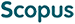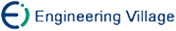Li Zhe, Li Zhanshan, Li Ying. A Constraint Network Model and Parallel Arc Consistency Algorithms Based on GPU[J]. Journal of Computer Research and Development, 2017, 54(3): 514-528. doi: 10.7544/issn1000-1239.2017.20150912
 Citation: Li Zhe, Li Zhanshan, Li Ying. A Constraint Network Model and Parallel Arc Consistency Algorithms Based on GPU[J]. Journal of Computer Research and Development, 2017, 54(3): 514-528. doi: 10.7544/issn1000-1239.2017.20150912# A Constraint Network Model and Parallel Arc Consistency Algorithms Based on GPU• Constraint satisfaction problem is a popular paradigm to deal with combinatorial problems in artificial intelligence. Arc consistency (AC) is one of basic solution compression algorithms of constraint satisfaction problem, which is also a core algorithm of many excellent advanced algorithms. When constraints are considered independently, AC corresponds to the strongest form of local reasoning. An effective underlying AC can improve the efficiency of reducing the search space. Recent years, GPU has been used for constituting many super computers, which solve many problems in parallel. Based on GPU's computation, this paper proposes a constraint networks presentation model N-E and its parallel generation algorithm BuildNE. According to fine-grained arc consistency AC4, a parallel edition AC4\+GPU and its improved algorithm—AC4\+GPU, are proposed. The two parallel algorithms extend arc consistency to GPU. Experimental results verify the feasibility of these new algorithms. Compared with AC4, the parallel versions have made the 10% to 50% acceleration in some smaller instances, and obtained 1 to 2 orders of magnitude in some bigger instances. They provide a core algorithm to other constraint satisfaction problem solving in parallel for further study.
•/DownLoad:  Full-Size Img  PowerPoint